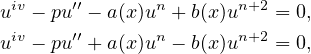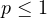Electron. J. Differential Equations, Vol. 2019 (2019), No. 119, pp. 1-14.

### Existence and multiplicity of positive periodic solutions for fourth-order nonlinear differential equations Hujun Yang, Xiaoling Han

Abstract:
In this article we study the existence and multiplicity of positive periodic solutions for two classes of non-autonomous fourth-order nonlinear ordinary differential equationswhereis a positive integer,, and a(x),b(x) are continuous positive T-periodic functions. These equations include particular cases of the extended Fisher-Kolmogorov equations and the Swift-Hohenberg equations. By using Mawhin's continuation theorem, we obtain two multiplicity results these equations.

Submitted October 17, 2019. Published November 14, 2019.
Math Subject Classifications: 34C25, 34G20, 35A01.
Key Words: Fourth-order nonlinear differential equations; multiplicity; positive periodic solutions; Mawhin's continuation theorem.

Show me the PDF file (325 KB), TEX file for this article.Hujun Yang School of Mathematics and Statistics Northwest Normal University Lanzhou, 730070, China email: 982047468@qq.com Xiaoling Han School of Mathematics and Statistics Northwest Normal University Lanzhou, 730070, China email: hanxiaoling@nwnu.edu.cn